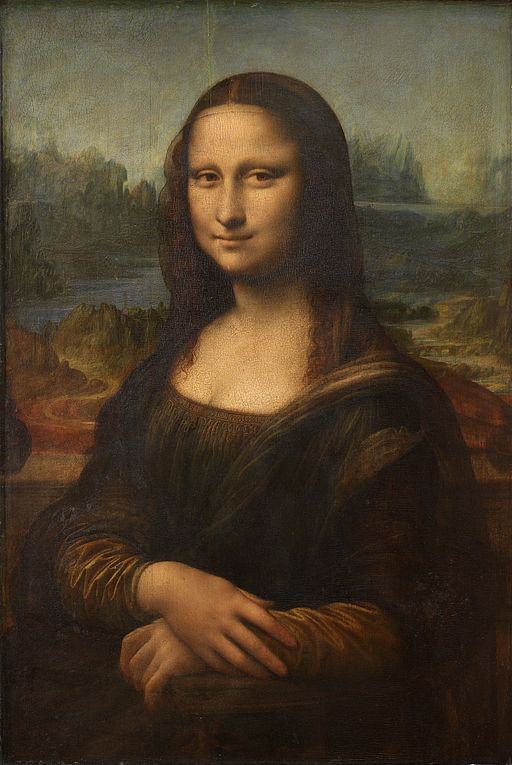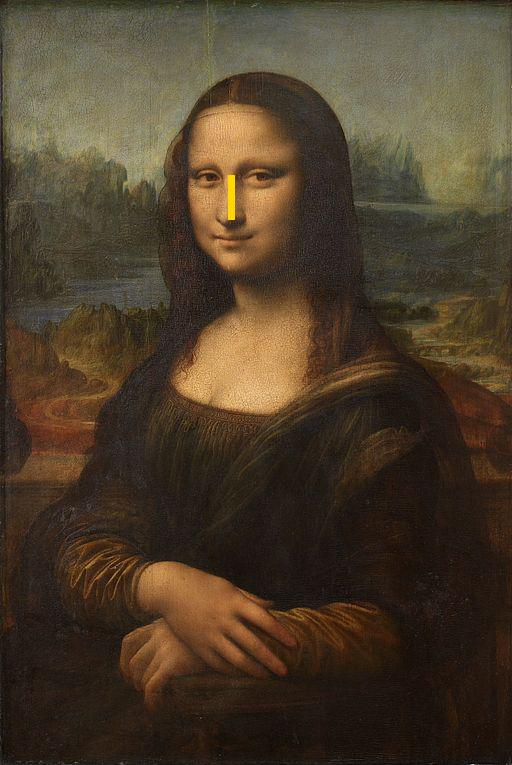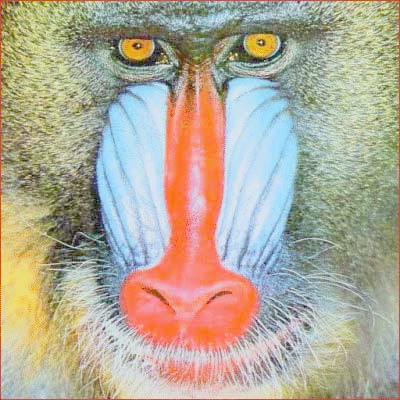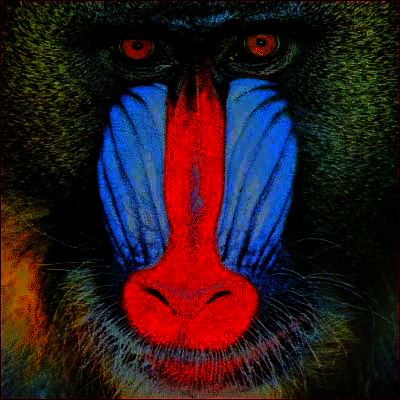## Class 37 April 18

#### Image manipulation

I need a haiku — I cannot find one I like — Can you help me please

### Module gamma.py

• Defines three functions in support of gamma correction, where a gamma correction supports the lightening and darkening of an image.

### Consider

#### Function `correction( i, gf )`

• Returns the gamma correction for gamma factor `gf` for value intensity `i`; that is, it returns the integer `i_prime` equal to

`int( 255 ( i / 255 )` `1/gf`  `)`

#### Function `pixel( p, gf )`

• Returns a new pixel whose RGB values are gamma corrections for gamma factor `gf` of the RGB values of pixel `p`. That is, if pixel `p` RGB values are `(r, g, b)`, then new pixel has RGB values `(correction(r, gf), correction(g, gf), correction(b,` `gf))`

#### Function `create( original, gf )`

• Returns a new image whose dimensions match that of `original`, where a pixel in the new image is a gamma factor `gf` correction of the corresponding pixel in `original`.

### To do

• Homework 26 — a bottom-up assignment### Imaging effects — gammaOriginal Gamma = 2.0 Gamma = 0.25

### Homework motivation